EXPERIMENT NO. :7

Study of Broadside array antenna

Practical Session Plan

 Time ( min) Content Learning Aid / Methodology Faculty Approach Typical Student Activity Skill / Competency Developed 10 Relevance and significance of experiment Chalk & Talk , Presentation Introduces, Facilitates, Monitors Listens, Participates, Discusses Knowledge, Communication, intrapersonal 20 Explanation of experiment Chalk & Talk , Presentation Introduces, Facilitates, Explains Listens Knowledge, Communication, intrapersonal, Application 10 Calculations N/A Explains, Monitors Participates, Discusses Knowledge, Communication, Intrapersonal, Application 50 Programming Demonstration Explains, Monitors Participates, Discusses Debate, comprehension, Hands on experiment 10 Debugging & Simulating Demonstration Explains, Monitors Participates, Discusses Hands on experiment 10 Results and conclusions Keywords Lists, Facilitates Listens, Participates, Discusses Knowledge, Communication, intrapersonal, Comprehension 10 Oral Question Discussion Discussion Discussion Identifies & Participates Knowledge Intrapersonal

Title: Study of Broadside array antenna.

Objectives: Model the Broad side Array to analyze the performance parameters

Aim:

Â· To sketch the radiation pattern of Broad side Array with uniform spacing & amplitude

Â· To compute performance parameters

Apparatus: Matlab software, PC, Printer.

Theory:

Broadside array is one in which number of identical parallel antennas are set up along a line drawn perpendicular to their respective axes as shown in fig 1(a).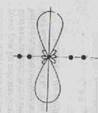Fig. 1 (a) Broadside array arrangement (b) Radiation pattern

In broadside array, individual antennas are equally spaced along a line and each element is fed with equal magnitude, all in same phase. This arrangement fires in broadside directions i.e. perpendicular to the line of array axis, there are maximum radiations and relatively a little radiations in other directions and hence the radiation pattern of broadside array is bidirectional as shown in fig 1(b)., which radiates equally well in either direction of maximum radiations.

In broadside array, it is desired to have maximum radiation of an array directed normal to the axis of the array (broadside=90Â°) to optimize the design, the maxima of single element can be accomplished by the choice of the radiators and those of array factor by the proper separation and excitation of the individual radiators.

For n-element linear array with uniform amplitude and spacing normalised Array factor is given as:â€¦â€¦â€¦â€¦.. (1)

Maximum Array factor occurs whenSince, it is desired to have the maximum directed toward Î¸ = 90Âº, then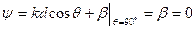To have maximum of AF directed broadside to the axis of array, it is necessary that all the elements have same phase excitation. The separation between the elements can be of any value. To ensure that there are no maximum in other directions, the separation between the elements should not be equal to multiples of a wavelength (d â‰  nÎ», n=1,2,3â€¦â€¦) when Î²=0. If d =nÎ», n=1,2,3â€¦â€¦ and Î² = 0, then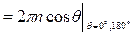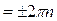This value of Ïˆ, when substituted in equation 1 makes the array factor attain its maximum value.

ALGORITHM:

1. Read values of NUMBER OF ARRAY ELEMENT (N), FREQUENCY (F), DISTANCE

BETWEEN ELEMENTS (d)

2. Calculate WAVELENGTH Î» = C/F WITH (c=3*108m/sec).

3. Calculate Phase Ïˆ.

4. Plot polar graph Î¦ vs. AF.

5. Calculate non-normalized AFâ€™ = N*AF.

6. The calculate AF in dB scale. = AFdn

7. AFavg= (AFdn+ AFâ€™) / 2

8. Plot polar graph Î¦ vs. AFavg

9. Calculate FNBW, HPBW, FSLBW using given formulae.

Matlab Code For Broadside Array:-

clc;

lambda=0.1;

N=input('Enter the no of elements of the array');%N=10 elements arranged in array

d=0.5*lambda; %Spacing betweeen elements d=lamba/2

%d=input('Enter the spacing between the elements');

beta=0;%for broad side array beta= 0

k=2*pi/lambda;

theta=0:pi/180:2*pi; % theta indicatea that radiation pattern is in two direction i.e perpendicular to axisof

si=k.*d.*cos(theta)+beta;

AF=1/N *((sin(N.*si/2))./sin((si./2)));% array factor

plot(theta *180/pi,10*log10(abs(AF)));

xlabel('theta in degree')

ylabel('amplitude in db')

figure(2);

polar(theta,abs(AF));

figure(3);

U=(abs(AF)./max(abs(AF))).^2;% eliminating side lobes

polar(theta,U);

clc;

Oral Question Bank

Theory Question Bank

 Q. No Description 1 What is an â€œarray factorâ€? 2 What are the types of antenna arrays? 3 Define the length of an array 4 What are the applications of arrays? 5 How to convert broad side array radiation pattern into unidirectional? 6 Define antenna array. 7 What is an array and mention the various forms of antenna arrays? 8 What is array factor or space factor? 9 Define HPBW? 10 Define BWFN? 11 An array consisting a no. of equidistant dipoles of equal size, fed with the same currents and phase is called:(a) end fire array (b) back fire array (c) broad side array (d) binomial array 12 Define FSLBW? 13 What is a collinear array? 14 What are the characteristics of broad side array? 15 Which are the controlling parameters of the overall pattern of antenna array? 16 Why antenna arrays are required? 17 What is uniform linear array? 18 What is the effect of changing the number of element on the radiation pattern of an antenna array? 19 What is the effect of changing the spacing between adjacent elements on radiation pattern of an antenna array? 20 Write the principle of pattern multiplication?

Results:

Expected Matlab Output: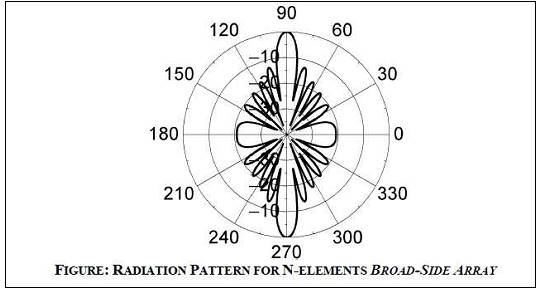Conclusion:

In BROAD SIDE ARRAY ANTENNA the maximum radiation is always perpendicular to the axis of antenna array i.e. at 90Âº and 270Âº and the maximum radiation can be obtained by increasing the number of elements.

Upon completion of experiment students will be able to:

Students will able to model the Broadside array antenna, compute the performance parameters and compare the performance parameters with standard parameters.